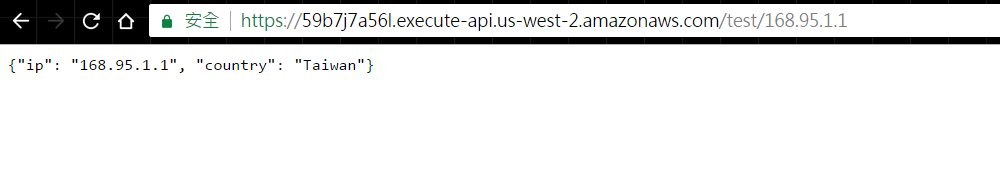SELECT *
FROM ip_geo_table
WHERE IP_START <= '8.8.8.8' AND
IP_END >= '8.8.8.8'

簡化問題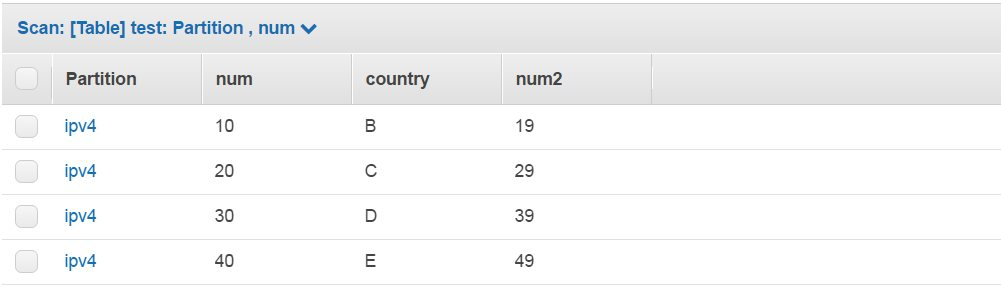Query條件設為: partition = 'ipv4' , num1 <=25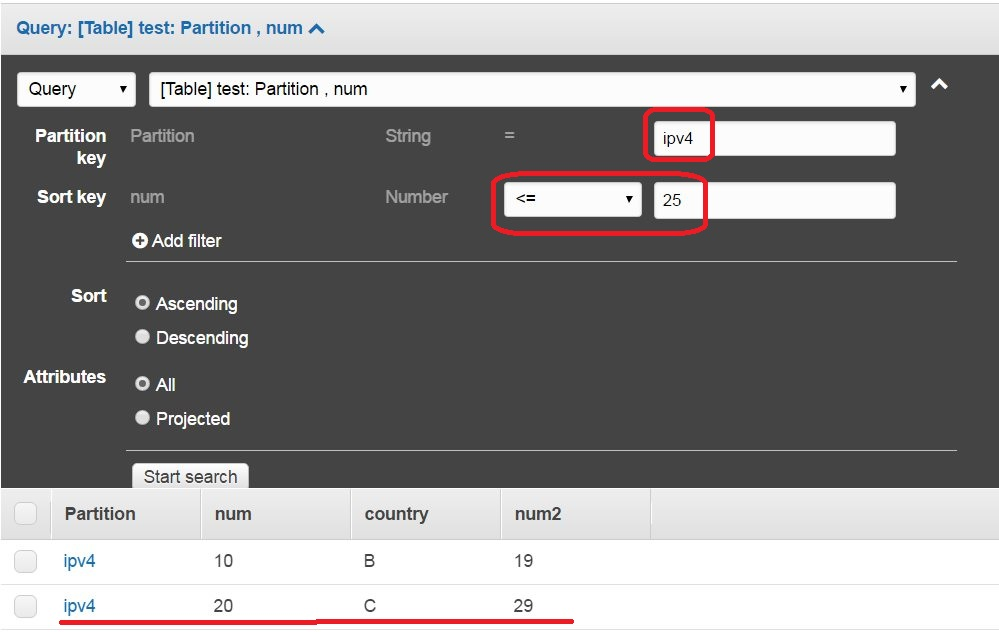Query條件設為: partition = 'ipv4' , num1 <=25 ,排序: 倒著排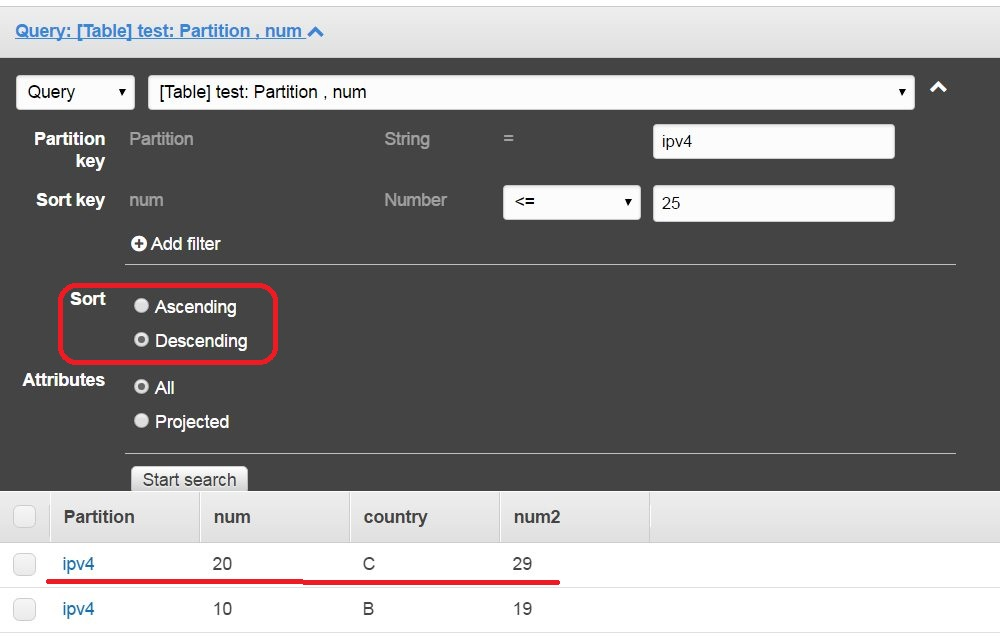查詢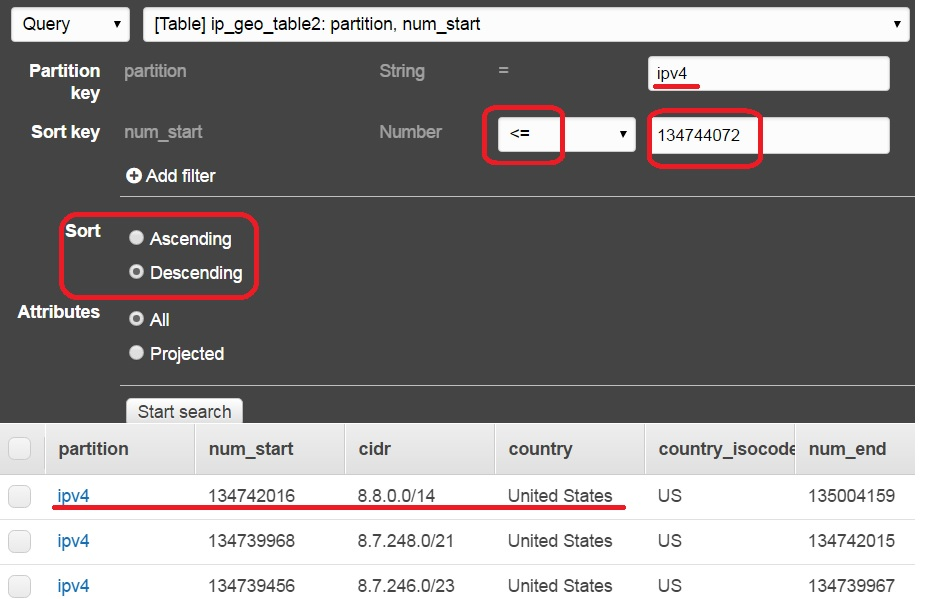盲點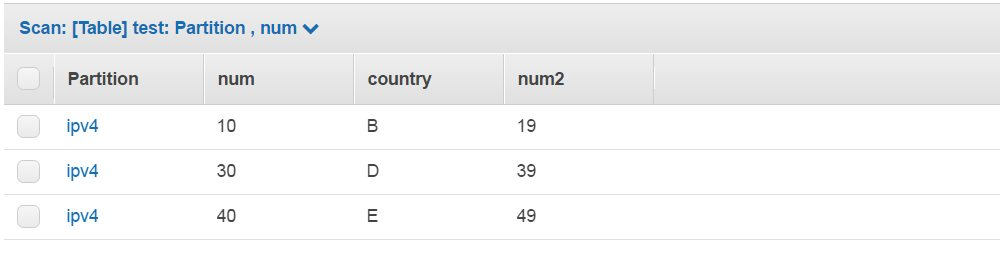151.219.168.215
102.105.233.217
154.169.7.126
103.177.49.97

Python Query DynamoDB 範例

import socket
import struct
import boto3
from boto3.dynamodb.conditions import Key

DYNAMODB = boto3.resource('dynamodb', region_name='us-west-2')
IPGEO_TABLE = DYNAMODB.Table('ip_geo_table')

def main():

country = dynamodb_query('8.8.8.8')
print(country)

'''ip to int
'''

def dynamodb_query(s_ip):
'''Query
'''
the_ip = ip2int(s_ip)

response = IPGEO_TABLE.query(
KeyConditionExpression=Key('partition').eq(
'ipv4') & Key('num_start').lte(the_ip),
ScanIndexForward=False,
Limit=1
)

return response

搭配Lambda + API Gateway服務